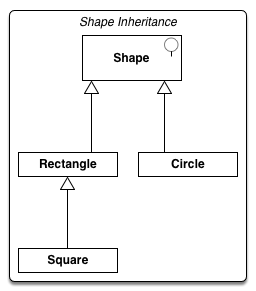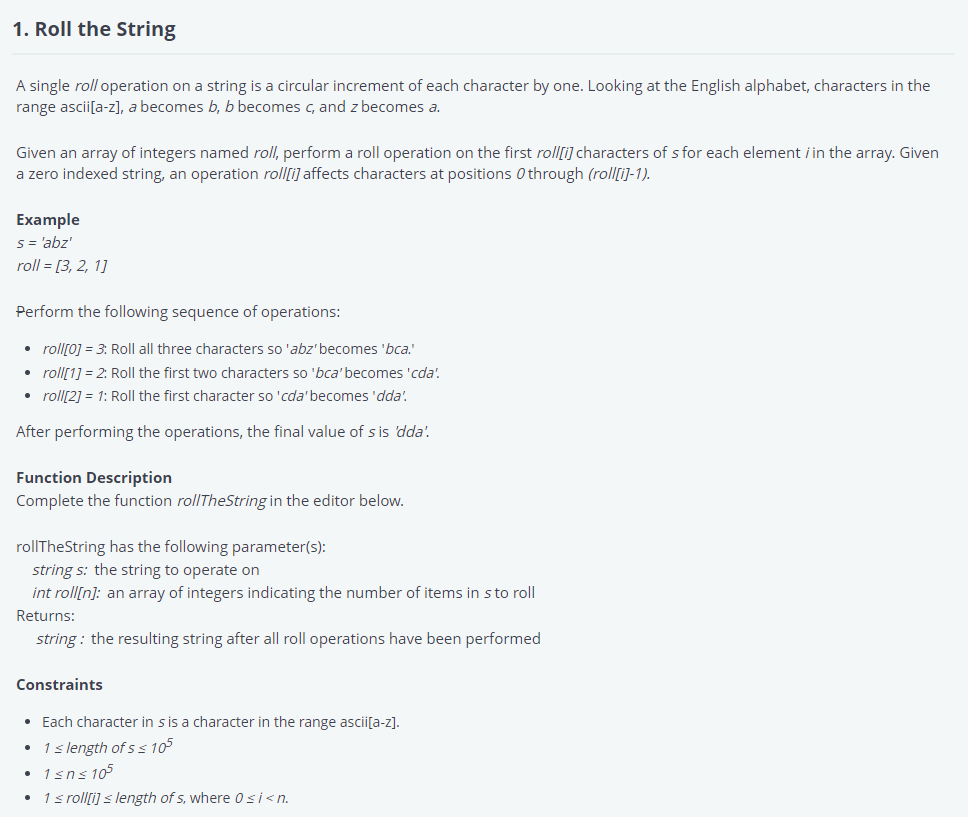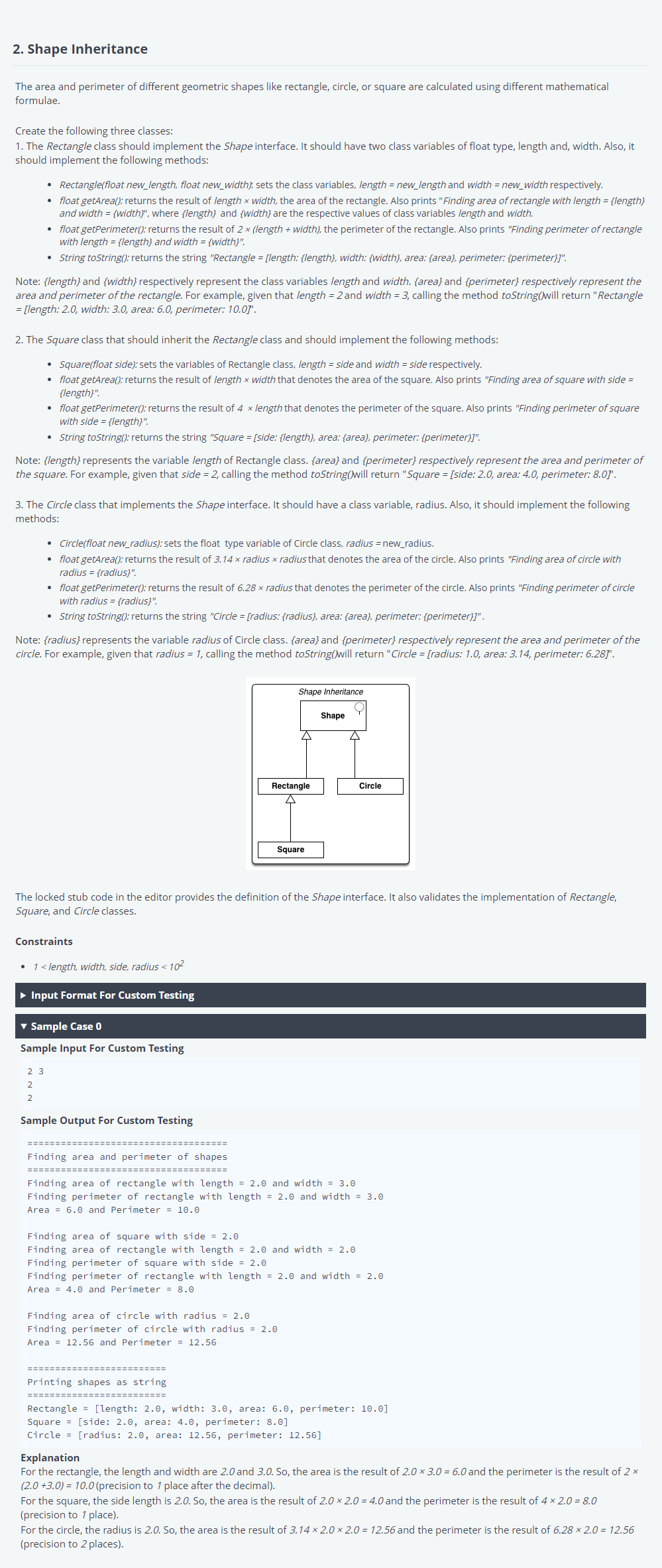folder_open

arrow_right
article

# 面試心得–Migo 熱鬧點科技

2021/09/30~2021/10/06【Senior Backend Engineer】未錄取

# 第二輪線上技術測驗#

## 1. Roll The String#

A single roll operation on a string is a circular increment of each character by one. Looking at the English alphabet, characters in the range ascii[a-z], a becomes b, b becomes c, and z becomes a.

Given an array of integers named roll, perform a roll operation on the first roll[i] characters of s for each element i in the array. Given a zero indexed string, an operation roll[i] affects characters at positions 0 through (roll[i]-1).

Example

s = 'abz'

roll = [3, 2, 1]

Perform the following sequence of operations:

• roll = 3: Roll all three characters so 'abz' becomes 'bca'.
• roll = 2: Roll the first two characters so 'bca' becomes 'cda'.
• roll = 1: Roll the first character so 'cda' becomes 'dda'.

After performing the operations, the final value of s is 'dda'.

Function Description

Complete the function rollTheString in the editor below.

rollTheString has the following parameter(s):

• string s: the string to operate on
• int roll[n]: an array of integers indicating the number of items in s to roll

Returns:

• string: the resulting string after all roll operations have been performed

Constraints

• Each character in s is a character in the range ascii[a-z].
• $1 \leqslant$ length of s $\leqslant 10^5$
• $1 \leqslant n \leqslant 10^5$
• $1 \leqslant$ roll[i] $\leqslant$ length of s, where $0 \leqslant i \lt n$.

## 2. Shape Inheritance#

The area and perimeter of different geometric shapes like rectangle, circle, or square are calculated using different mathematical formulae.

Create the following three classes:

1. The Rectangle class should implement the Shape interface. It should have two class variables of float type, length and, width. Also, it should implement the following methods:

• Rectangle(float new_length, float new_width): sets the class variables, length = new_length and width = new_width respectively.
• float getArea(): returns the result of length $\times$ width, the area of the rectangle. Also prints "Finding area of rectangle with length = {length} and width = {width}", where {length} and {width} are the respective values of class variables length and width.
• float getPerimeter(): returns the result of 2 $\times$ (length + width), the perimeter of the rectangle. Also prints "Finding perimeter of rectangle with length = {length} and width = {width}".
• String toString(): returns the string "Rectangle = [length: {length}, width: {width}, area: {area}, perimeter: {perimeter}]".

Note: {length} and {width} respectively represent the class variables length and width. {area} and {perimeter} respectively represent the area and perimeter of the rectangle. For example, given that length = 2 and width = 3, calling the method toString() will return "Rectangle = [length: 2.0, width: 3.0, area: 6.0, perimeter: 10.0]".

2. The Square class that should inherit the Rectangle class and should implement the following methods:

• Square(float side): sets the variables of Rectangle class, length = side and width = side respectively.
• float getArea(): returns the result of length $\times$ width that denotes the area of the square. Also prints "Finding area of square with side = {length}"
• float getPerimeter(): returns the result of 4 $\times$ length that denotes the perimeter of the square. Also prints "Finding perimeter of square with side = {length}"
• String toString(): returns the string "Square = [side: {length}, area: {area}, perimeter: {perimeter}]".

Note: {length} represents the variable length of Rectangle class. {area} and {perimeter} respectively represent the area and perimeter of the square. For example, given that side = 2, calling the method toString() will return "Square = [side: 2.0, area: 4.0, perimeter: 8.0]".

3. The Circle class that implements the Shape interface. It should have a class variable, radius. Also, it should implement the following methods:

• float getArea(): returns the result of 3.14 $\times$ radius $\times$ radius that denotes the area of the circle. Also prints "Finding area of circle with radius = {radius}".
• float getPerimeter(): returns the result of 6.28 $\times$ radius that denotes the perimeter of the circle. Also prints "Finding perimeter of circle with radius = {radius}".
• String toString(): returns the string "Circle = [radius: {radius}, area: {area}, perimeter: {perimeter}]".

Note: {radius} represents the variable radius of Circle class. {area} and {perimeter} respectively represent the area and perimeter of the circle. For example, given that radius = 1, calling the method toString() will return "Circle = [radius: 1.0, area: 3.14, perimeter: 6.28]".The locked stub code in the editor provides the definition of the Shape interface. It also validates the implementation of Rectangle, Square, and Circle classes.

Constraints

• $1 \lt$ length, width, side, radius $\lt 10^2$

# 面試結果及時程#

• 2021/09/30 第一輪人資電話面試
• 2021/10/05 第二輪線上技術測驗
• 2021/10/06 感謝信

# 附錄：第二輪線上技術測驗題目參考解答#

## 1. Roll The String##!/bin/python3
import mathimport osimport randomimport reimport sys
## Complete the 'rollTheString' function below.## The function is expected to return a STRING.# The function accepts following parameters:#  1. STRING s#  2. INTEGER_ARRAY roll#
def rollTheString(s, rolls):    n = len(s)    roll_counts =  * n
alphbets = 'abcdefghijklmnopqrstuvwxyz'    alphbet_map = {a: i for i, a in enumerate(alphbets)}
for roll in rolls:        for i in range(roll):            roll_counts[i] += 1
results = []    for i, roll_count in enumerate(roll_counts):        original_alphabet = s[i]        k = alphbet_map[original_alphabet]        rolled_alphabet = alphbets[(k + roll_count) % 26]        results.append(rolled_alphabet)
return ''.join(results)
if __name__ == '__main__':    # ...

def rollTheString(s, rolls):    s_len = len(s)    rolls_len = len(rolls)    roll_counts =  * s_len
for roll in rolls:        roll_counts[roll - 1] += 1
for i in range(rolls_len - 2, -1, -1):        roll_counts[i] += roll_counts[i + 1]
alphbets = 'abcdefghijklmnopqrstuvwxyz'    alphbet_map = {a: i for i, a in enumerate(alphbets)}
results = []    for i, roll_count in enumerate(roll_counts):        original_alphabet = s[i]        k = alphbet_map[original_alphabet]        rolled_alphabet = alphbets[(k + roll_count) % 26]        results.append(rolled_alphabet)
return ''.join(results)
assert rollTheString('a', ) == 'b'assert rollTheString('aa', [1, 1]) == 'ca'assert rollTheString('bca', [1, 2, 3]) == 'eeb'assert rollTheString('zcza', [1, 1, 3, 4]) == 'debb'

## 2. Shape Inheritance#import java.util.Scanner;
interface Shape {    float getArea();    float getPerimeter();    String toString();}
/* * Write the implementations of the Rectangle, Square, and Circle classes. */
public class Solution {    private static final Scanner INPUT_READER = new Scanner(System.in);
public static void main(String[] args) {        String[] lengthWidth = INPUT_READER.nextLine().split(" ");        float length = Float.parseFloat(lengthWidth);        float width = Float.parseFloat(lengthWidth);
Shape rectangle = new Rectangle(length, width);        Rectangle square = new Square(side);        Shape circle = new Circle(radius);
System.out.println("====================================");        System.out.println("Finding area and perimeter of shapes");        System.out.println("====================================");
System.out.println("Area = " + rectangle.getArea() + " and Perimeter = " + rectangle.getPerimeter() + "\n");        System.out.println("Area = " + square.getArea() + " and Perimeter = " + square.getPerimeter() + "\n");        System.out.println("Area = " + circle.getArea() + " and Perimeter = " + circle.getPerimeter() + "\n");
System.out.println("=========================");        System.out.println("Printing shapes as string");        System.out.println("=========================");
System.out.println(rectangle.toString());        System.out.println(square.toString());        System.out.println(circle.toString());    }
public static class Rectangle implements Shape {        float length;        float width;
public Rectangle(float new_length, float new_width) {            this.length = new_length;            this.width = new_width;
}
@Override        public float getArea() {            System.out.println("Finding area of rectangle with length = " + this.length + " and width = " + this.width);            return this._getArea();        }
@Override        public float getPerimeter() {            System.out.println("Finding perimeter of rectangle with length = " + this.length + " and width = " + this.width);            return _getPerimeter();        }
@Override        public String toString() {            return "Rectangle = [length: " + this.length + ", width: " + this.width + ", area: " + _getArea() + ", perimeter: " + _getPerimeter() + "]";        }
protected float _getArea() {            return this.length * this.width;        }
protected float _getPerimeter() {            return (this.length + this.width) * 2;        }    }
public static class Square extends Rectangle {        public Square(float side) {            super(side, side);        }
@Override        public float getArea() {            System.out.println("Finding area of square with side = " + this.length);            return super.getArea();        }
@Override        public float getPerimeter() {            System.out.println("Finding perimeter of square with side = " + this.length);            return super.getPerimeter();        }
@Override        public String toString() {            return "Square = [side: " + this.length + ", area: " + _getArea() + ", perimeter: " + _getPerimeter() + "]";        }    }
public static class Circle implements Shape {        float radius;
protected float _getPerimeter() {            return (float)6.28 * this.radius;        }    }